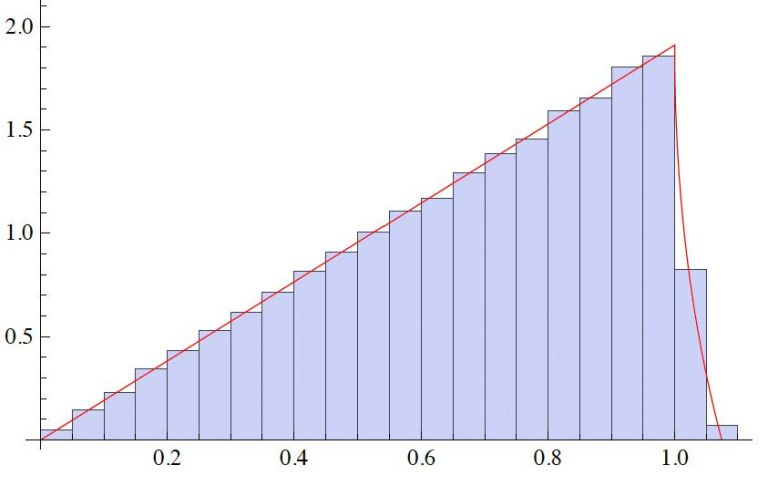# Lattice reduction and invariant measure## lattice_reduction_and_invariant_measure.jpg

Comparison of numerically generated distribution for the magnitude of the shortest lattice vector in two dimensions for a basis chosen with invariant measure, and the theoretic prediction.

Lattices are the span of linearly independent vectors over the integers. Lattice reduction seeks to find a basis of shortest vectors in the lattice. This objective has applications in cryptography, coding theory, the global positioning system, and statistical signal processing, among other examples. Only up to dimension four is there a polynomial time algorithm to find shortest lattice vectors. In dimension two, this is classical and due to Lagrange and Gauss. A celebrated approximate algorithm is due to Lenstra, Lenstra and Lovasz (LLL). There is a unique invariant measure on the space of lattices, and part of this project is to formulate practical methods to sample from this. Lattice reduction to a fundamental domain allows histograms approximating the probability density functions of the lengths and pairwise angles of the shortest basis vectors for dimensions two and three, and in dimension two these distributions can be evaluated explicity. Together with MSc student Jiyuan Zhang, an analogous study in the case of complex lattices with spanning set the Gaussian integers is presently being studied.